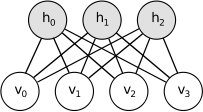# 基于能量的模型和波尔兹曼机

## 1. 基于能量的模型(EBM)

p(x)=eE(x)Z. … ①

Z=xeE(x). … ②

l(θ,D)=L(θ,D)=1Nx(i)Dlogp(x(i)). … ③

Δ=l(θ,D)θ=1Nlogp(x(i))θ. … ④

### 包含隐单元的EBMs

P(x)=hP(x,h)=heE(x,h)Z. … ⑤

F(x)=logheE(x,h). … ⑥

P(x)=eF(x)Z,whereZ=xeF(x). … ⑦

Δ=logp(x)θ=(F(x)logZ)θ=F(x)θx^p(x^)F(x^)θ. … ⑧

## 2. 限制性玻尔兹曼机RBM的能量函数E(v,h)定义为，
E(v,h)=bvchhWv.

F(v)=bviloghiehi(ci+Wiv). … ⑨

p(h|v)=ip(hi|v),
p(v|h)=jp(vj|h). … ⑩

### 使用二值单元的RBM

p(hi=1|v)=sigmoid(ci+Wiv),
p(vj=1|h)=sigmoid(bj+Wjh). … ⑪

F(v)=bvilog(1+eci+Wiv). … ⑫

logp(v)Wij=Ev[p(hi|v)vj]v(i)jsigmoid(Wiv(i)+ci),
logp(v)ci=Ev[p(hi|v)vj]sigmoid(Wiv(i)),
logp(v)bj=Ev[p(hi|v)vj]v(i)j. … ⑬

## 3. RBM的学习

### 3.1 Gibbs采样

Gibbs采样是一种基于马尔科夫链蒙特卡罗(Markov Chain Monte Carlo,MCMC)策略的采样方法。对于一个K为随机向量X=(X1,X2,,XK)， 假设我们无法求得关于X的联合分布P(X)，但我们知道给定X的其他分量时，其第k个分量Xk的条件分布，即P(Xk|Xk)，其中Xk=(X1,X2,,Xk1,Xk+1,,XK)，那么，我们可以从X的一个任意状态(比如[x1(0),x2(0),,xK(0)])开始，利用上述条件 分布，迭代的对其分量依次采样，随着采样次数的增加，随机变量[x1(n),x2(n),,xK(n)]的概率分布将以n的几何级数的速度收敛于X的联合 概率分布P(X)。也就是说，我们可以在未知联合概率分布的条件下对其进行采样。

h0P(h|v0),v1P(v|h0),
h1P(h|v1),v2P(v|h1),
,vk+1P(v|hk).

### 3.2 对比散度算法

 1 2 3 4 5 6 7 8 9 10 11 12 13 14 15 16 17 18 19 20 21 22 23 24  //输入：一个训练样本x0; 隐层单元个数m; 学习率alpha; 最大训练周期T //输出：链接权重矩阵W, 可见层的偏置向量a, 隐层的偏置向量b //训练阶段 初始化：令可见层单元的初始状态v1 = x0; W, a, b为随机的较小数值 For t=1,2,...,T For j=1,2,...,m //对所有隐单元 计算P(h1j=1|v1), 即P(h1j=1|v1) = sigmoid(bj+sum_i(v1i*Wij)); 从条件分布P(h1j|v1)中抽取h1j ∈ {0,1} EndFor For i=1,2,...,n //对所有可见单元 计算P(v2i=1|h1), 即P(v2i=1|h1) = sigmoid(ai+sum_j(Wij*h1j)); 从条件分布P(v2i|h1)中抽取v2i ∈ {0,1} EndFor For j=1,2,...,m //对所有隐单元 计算P(h2j=1|v2), 即P(h2j=1|v2) = sigmoid(bj+sum_i(v2i*Wij)); EndFor //更新RBM的参数 W = W + alpha *(P(h1=1|v1)v1' - P(h2=1|v2)v2'); a = a + alpha *(v1-v2); b = b + alpha *(P(h1=1|v1) - P(h2=1|v2)); EndFor

RBM的完整实现参见https://github.com/ibillxia/DeepLearnToolbox/tree/master/DBN的Matlab代码。

## References

 Learn Deep Architectures for AI, Chapter 5.
 Deep Learning Tutorial, Release 0.1, Chapter 9.
 受限波尔兹曼机简介. 张春霞.
 Training Products of experts by minimizing contrastive divergence. GE Hinton.